### Real Vim ninjas count every keystroke - do you?

###### Pick a challenge, fire up Vim, and show us what you got.

```Your VimGolf key: please sign in

\$ gem install vimgolf
\$ vimgolf setup
\$ vimgolf put 5d78aa6071f751000cfc0bfc
```

### calculations

Complete these (weird) calculations.

##### Start file
```5+11+one=
8*2+one=
100+99+one=
123+123+one=
4+4+two=
12*9+two=
1+1+one=
one+one+one+one=
two*8=
two+two+one+two=
two*two*two*two*two*two*two*two=
two*two*two*two*two*two*two*two*two*two*two*two*two*two*two*two=
```
##### End file
```5+11+one=17
8*2+one=17
100+99+one=200
123+123+one=247
4+4+two=10
12*9+two=110
1+1+one=3
one+one+one+one=4
two*8=16
two+two+one+two=7
two*two*two*two*two*two*two*two=256
two*two*two*two*two*two*two*two*two*two*two*two*two*two*two*two=65536
```

#### View Diff

```1,12c1,12
< 5+11+one=
< 8*2+one=
< 100+99+one=
< 123+123+one=
< 4+4+two=
< 12*9+two=
< 1+1+one=
< one+one+one+one=
< two*8=
< two+two+one+two=
< two*two*two*two*two*two*two*two=
< two*two*two*two*two*two*two*two*two*two*two*two*two*two*two*two=
---
> 5+11+one=17
> 8*2+one=17
> 100+99+one=200
> 123+123+one=247
> 4+4+two=10
> 12*9+two=110
> 1+1+one=3
> one+one+one+one=4
> two*8=16
> two+two+one+two=7
> two*two*two*two*two*two*two*two=256
> two*two*two*two*two*two*two*two*two*two*two*two*two*two*two*two=65536
```

### Solutions

The best way to learn is to practice. Below, you will find some of the solutions other golfers have entered. To unlock higher ranked solutions, submit your own entry which does as well or better than the solutions you can currently see - climb the ladder!

Check out these helpful resources to improve your Vim skills... Game on.

Unlock 63 remaining solutions by signing in and submitting your own entry
###### #64 huynhminhchu / @huynhminhchu - Score: 183 - 09/22/19 @ 08:44
```A<C-R>=5+11+1<CR><Esc>jA<C-R>=8*2+1<CR>j<BS><Esc>jA<C-R>=100+99+1<CR><Esc>jA<C-R>=123+123+1<CR><Esc>jA<C-R>=4+4+2<CR><Esc>j.xxA<C-R>=12*9+2<CR>j<BS><Esc>jA<C-R>=1*3<CR><Esc>jA<C-R>=1*4<CR>j<BS><Esc>jA<C-R>=2*8<CR><Esc>jA<C-R>=2*3+2<BS>1<CR><Esc>jA<C-R>=2**8<CR>2<BS><C-R>=2*2*2*2*2*2*2*2<CR><Esc>jA<C-R><C-R>=256*2*2*2*2*2*2*2*2<CR><Esc>:wq<CR>
```

## 64 active golfers, 262 entries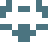38
###### #1 - Saki Gw / @Sakigw

09/11/2019 at 09:22AM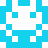38
###### #2 - John Braxler / @braxler

09/11/2019 at 12:41PM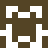38
###### #3 - Smylers / @Smylers2

09/13/2019 at 10:28AM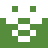38
###### #4 - Douglas Massolari / @dmassolari

09/16/2019 at 03:57PM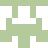38
###### #5 - Cassandra / @kascein

09/17/2019 at 06:20AM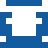38
###### #6 - Viktor Styrud / @ViktorStyrud

09/20/2019 at 02:49PM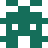38
###### #7 - vimgolfer / @vimgolfer7

09/21/2019 at 03:11PM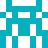38
###### #8 - Paul Yates / @PYates77

10/18/2019 at 09:15PM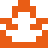38
###### #9 - Tamás Kárándi / @tkarandi

10/20/2019 at 07:40AM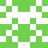38
###### #10 - Ivan Volosyuk / @IvanVolosyuk

10/22/2019 at 04:10AM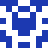38
###### #11 - floyd / @floyd10086

04/06/2020 at 10:12AM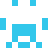38
###### #12 - Jung Yeon Park / @park_jungy

02/06/2021 at 10:46PM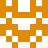38
###### #13 - hamb / @hamb88140219

03/05/2021 at 08:09AM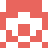38
###### #14 - pikjes / @pikjes2

03/21/2021 at 06:57PM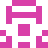38
###### #15 - Roman Privalov / @RomanPrivalov

09/21/2021 at 04:44PM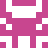38
###### #16 - }(⇒){ / @TangerinePlank

06/18/2022 at 05:21AM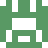38
###### #17 - Luoyb / @Luoyb

10/27/2023 at 07:48AM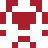39
###### #18 - Baine Wedlock / @BaineWedlock

09/11/2019 at 01:50PM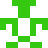39
###### #19 - asfsef / @asfsef5

09/11/2019 at 09:38PM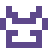39
###### #20 - Tosiaki / @Tosiaki1

09/15/2019 at 06:20PM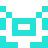39
###### #21 - Roger Stoltz / @rogsto1

11/14/2019 at 11:32PM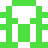39
###### #22 - JimmyGymglish / @JGymglish

01/02/2020 at 02:18PM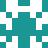39
###### #23 - garry quested / @GQuested

02/18/2020 at 10:45PM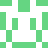39
###### #24 - chocolate / @chocola81394123

11/01/2020 at 09:56AM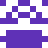39
###### #25 - hepebexè / @hepebexe

02/07/2021 at 03:00PM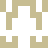39
###### #26 - Jianfeng / @Jianfen22425058

02/08/2021 at 08:45AM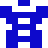40
###### #27 - theBecwar / @thebecwar

09/12/2019 at 12:50AM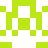40
###### #28 - Simon / @SimontheLeg

09/13/2019 at 02:38PM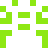40
###### #29 - Mario Olofo / @mario_olofo

10/19/2019 at 11:45PM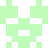40
###### #30 - yousef Ibrahim / @YucefIbrahim

10/25/2019 at 01:03PM